• 本文档包含了MATLAB遗传算法求解MTSP问题的代码 代码中有详细的注释 注意一点，本文来源网络，侵删！！！
• 5种非常好的MTSP求解MATLAB代码 每种代码注释非常详细
• 遗传算法解决5种多旅行商问题（mtsp）的matlab程序 分别为以下5中情况： 1.从不同起点出发回到起点（固定旅行商数量） 2.从不同起点出发回到起点（旅行商数量根据计算可变） 3.从同一起点出发回到起点 4.从同一起点...
• 本算法可以求得从一个城市出发的多路旅行商问题，而且通过参数设定，可使各路均衡，望对大家有所帮助。 本算法可以求得从一个城市出发的多路旅行商问题，而且通过参数设定，可使各路均衡，望对大家有所帮助。
• 遗传算法解决5种多旅行商问题（mtsp）的matlab程序 分别为以下5中情况： 1.从不同起点出发回到起点（固定旅行商数量） 2.从不同起点出发回到起点（旅行商数量根据计算可变） 3.从同一起点出发回到起点 4.从同一起点...
• 本文件提供了一个MTSP类，一个启动main函数，主要提供了几个可改参数，参数1：交叉概率；参数2：变异概率；参数3：种群数目； 参数4：迭代次数；参数5：旅行商的数量（根据实际情况调整）；参数6：每辆车最少去几个...
•Python
• 5种多旅行商问题(MTSP)的遗传算法 5种多旅行商问题(MTSP)的遗传算法
• mtsp的MATLAB代码，很难找，可用于解决多旅行商问题------------------------------
• 遗传算法解决5种多旅行商问题（mtsp）的matlab程序 分别为以下5中情况： 1.从不同起点出发回到起点（固定旅行商数量） 2.从不同起点出发回到起点（旅行商数量根据计算可变） 3.从同一起点出发回到起点 4.从同一起点...
• MTSP_GA_MULTI_CH 多重旅行推销员问题 (M-TSP) 使用多染色体表示的遗传算法 (GA) 通过设置找到 M-TSP 变体的（接近）最优解up a GA 搜索最短路径，考虑到额外的限制，并尽量减少销售人员的数量。 概括： 1. 每个销售...matlab
• 【路径规划】基于蚁群求解多旅行商MTSP问题matlab源码.md
• 遗传算法解决MTSP问题 个体的基因型： 基本算法： 代码及其解释 案例： 遗传算法代码： 代码结果 ​ 关于其他MTSP问题 同一起点同一终点： 随机起点随机终点 遗传算法解决MTSP问题 问题类型：解决所有...
目录
遗传算法解决MTSP问题
个体的基因型：
基本算法：
代码及其解释
案例：
遗传算法代码：
代码结果
​
关于其他MTSP问题
同一起点同一终点：
随机起点随机终点

遗传算法解决MTSP问题
问题类型：解决所有旅行商从同一地点出发，但不回到出发点，且各旅行商的终点不同。
个体的基因型：
一个个体的基因型包含两段：
1、路径基因型
2、中断点基因型
这些意味着什么呢？假设有3个旅行商，10个城市，城市代号1为起始点，那么假设遗传算法中产生了这么一个个体，他的基因型为：
路径基因型：[2 3 5 6 7 9 10 8]
中断点基因型：[2 4]
那么，这个个体代表的信息为：
旅行商1的旅游路径：1 2 3
旅行商2的旅游路径：1 5 6
旅行商3的旅游路径：1 7 9 10 8
基本算法：
1、设置5000个迭代次数，每一次迭代产生一个最佳个体，若这厮的路径距离小于历史的全局最小值，就作为全局最小值。
2、从本次迭代中的个体，随机分成n组，从每一组中的最佳个体里修改基因片段（有的改路径基因型，有的改中断点基因型），从而得到子代。
3、子代再一次产生最小路径值，若再次小于历史的最小值，则设置他为全局最小值。再次以2的方法产生子代
4、直到5000次迭代结束为止。

代码及其解释
案例：
35个城市，5个旅行商。找到最小路径。
n = 35;    %设置城市个数
xy = 10*rand(n,2);   %随机产生城市的坐标，实际应用中可以自己输入坐标。主要用以画图，真正起作用的是距离矩阵啦。
salesmen = 5;   %设置旅行商的人数
min_tour = 3;    %设置每个旅行商至少走过三个城市（除去起始点和终止点的话就是一个城市）
pop_size = 80;    %设置种群的个数，必须是8的倍数，因为代码中以 8 做为步骤 2 的分组个数
num_iter = 5e3;   %设置迭代总次数， i.e. 5000次
a = meshgrid(1:n);   %用以计算距离矩阵。
dmat = reshape(sqrt(sum((xy(a,:)-xy(a',:)).^2,2)),n,n);   %计算距离矩阵（欧式距离），可以自己输入。
[opt_rte,opt_brk,min_dist] = mtspof_ga(xy,dmat,salesmen,min_tour, ...
pop_size,num_iter,1,1);  %运行代码


遗传算法代码：
根据以上的基本步骤，写出代码如下（包括：检查输入是否合理、画图与统计。）
function varargout = mtspofs_ga(xy,dmat,salesmen,min_tour,pop_size,num_iter,show_prog,show_res)
% MTSPOFS_GA Fixed Start Open Multiple Traveling Salesmen Problem (M-TSP) Genetic Algorithm (GA)
%   Finds a (near) optimal solution to a variation of the "open" M-TSP by
%   setting up a GA to search for the shortest route (least distance needed
%   for each salesman to travel from the start location to unique individual
%   cities without returning to the starting location)
%
% Summary:
%     1. Each salesman starts at the first point, but travels to a unique
%        set of cities after that (and none of them close their loops by
%        returning to their starting points)
%     2. Except for the first, each city is visited by exactly one salesman
%
% Note: The Fixed Start is taken to be the first XY point
%
% Input:
%     xy (float)：城市坐标，可以 2 维或者 3 维。
%     dmat (float) 距离矩阵 N X N 维
%     salesman (scalar integer) 旅行商个数。
%     min_tour (scalar integer) 不包含起始点，每个旅行商最少应该 travel 的数量。
%     pop_size (scalar integer) 遗传算法中个体的数量。
%     num_iter (scalar integer) 算法一共迭代的次数
%     show_prog (scalar logical) 如果等于1，就将迭代过程画出来
%     show_res (scalar logical) 如果等于1，就将算法最后的结果展示出来
%
% Output:
%     opt_rte (integer array) 输出最优个体的路径基因型
%     opt_brk (integer array) 输出最优个体的中断点基因型
%     min_dist (scalar float) 最有个体代表的所有旅行商的距离之和。
%
%
% Author: Joseph Kirk
% Email: jdkirk630@gmail.com
% Release: 1.3
% Release Date: 6/2/09
% commenter: zhuo

%检测输入参数，如果某些参数没有输入，那么改段代码就自动帮你加了。
nargs = 8;
for k = nargin:nargs-1
switch k
case 0
xy = 10*rand(40,2);  % 设置城市坐标的默认值为 40 个，随机产生 2 维坐标
case 1
N = size(xy,1);      %设置城市数目 N = size(xy,1)
a = meshgrid(1:N);
dmat = reshape(sqrt(sum((xy(a,:)-xy(a',:)).^2,2)),N,N);  %产生相应的距离矩阵。
case 2
salesmen = 5;   %旅行商个数的默认值为 5 个。
case 3
min_tour = 2;   %默认每个旅行商至少走 2 个城市
case 4
pop_size = 80;
case 5
num_iter = 5e3;
case 6
show_prog = 1;
case 7
show_res = 1;
otherwise
end
end

%验证输入是否可行，验证原理为城市个数 N 是否和 距离矩阵的 size相等
[N,dims] = size(xy);
[nr,nc] = size(dmat);
if N ~= nr || N ~= nc
error('Invalid XY or DMAT inputs!')
end
n = N - 1; % 除去了起始点

% Sanity Checks    还是验证输入：可以不看
salesmen = max(1,min(n,round(real(salesmen(1)))));
%验证输入的旅行商个数是不是大于1，并且是整数，否则帮你四舍五入改了
min_tour = max(1,min(floor(n/salesmen),round(real(min_tour(1)))));
%验证输入的minTour是不是大于1，并且是整数，否则帮你四舍五入改了
pop_size = max(8,8*ceil(pop_size(1)/8));
%验证输入的个体数是否为8的整数（因为后面的分组8个为一组），否则帮你用ceil函数改了
num_iter = max(1,round(real(num_iter(1))));
%验证输入的迭代次数是否大于1，否则帮改了
show_prog = logical(show_prog(1));
%验证是否为1或0，不然帮你改了，下同。
show_res = logical(show_res(1));

num_brks = salesmen-1;   % 设置中断点的个数，下面可以不看。
dof = n - min_tour*salesmen;
for k = 2:num_brks
end

% Initialize the Populations
pop_rte = zeros(pop_size,n);          % 所有个体的路径基因型
pop_brk = zeros(pop_size,num_brks);   % 所有个体的中断点基因型
for k = 1:pop_size
pop_rte(k,:) = randperm(n)+1;    %初始化所有个体的路径基因型。
%这里为什么加 1 呢？因为我们 n=N-1=34 ，因此产生的路径基因型必须在[2，35]中产生。
pop_brk(k,:) = randbreaks();   %产生中断点，函数代码在下方（子函数）
end

% 选择画图时的颜色（不同旅行商的路径画在图上要用不同颜色呀）
clr = [1 0 0; 0 0 1; 0.67 0 1; 0 1 0; 1 0.5 0];
if salesmen > 5
clr = hsv(salesmen);
end

% Run the GA
global_min = Inf;
total_dist = zeros(1,pop_size);   %每个个体的总距离构成的 1X80 维的向量
dist_history = zeros(1,num_iter);   %每一次迭代个数中最优个体的总距离构成的 1X5000 维的向量。
tmp_pop_rte = zeros(8,n);   %暂时变量，用完就丢。用于产生新个体的，（路径的基因型）
tmp_pop_brk = zeros(8,num_brks);    %同上，用于产生新的中断点的基因型
new_pop_rte = zeros(pop_size,n);    %新生代的路径基因型，为一个 80X34 的矩阵，每一行代表每一个个体。
new_pop_brk = zeros(pop_size,num_brks); %同上，用于中断点基因型 80X4 的矩阵。
if show_prog
pfig = figure('Name','MTSPOFS_GA | Current Best Solution','Numbertitle','off');
end
for iter = 1:num_iter     %计算一次迭代过程中，每一个个体的总距离
for p = 1:pop_size
d = 0;
p_rte = pop_rte(p,:);
p_brk = pop_brk(p,:);
rng = [[1 p_brk+1];[p_brk n]]';
for s = 1:salesmen
d = d + dmat(1,p_rte(rng(s,1))); % 加上起点到第二个城市的距离

%计算路径中城市的总距离：
for k = rng(s,1):rng(s,2)-1
d = d + dmat(p_rte(k),p_rte(k+1));
end
end
total_dist(p) = d;  %将每一个个体的距离存入到矩阵中。
end

% Find the Best Route in the Population
[min_dist,index] = min(total_dist);   %找到最优个体（index）
dist_history(iter) = min_dist;   %将其总距离存入到历史矩阵中。
%下一步是比较当前最优与全局最优，如果比他还小，那么就替换全局最优。
%并更新当前的路径图。
if min_dist < global_min
global_min = min_dist;
opt_rte = pop_rte(index,:);
opt_brk = pop_brk(index,:);
rng = [[1 opt_brk+1];[opt_brk n]]';
%更新路径图。
if show_prog
% Plot the Best Route
figure(pfig);
for s = 1:salesmen
rte = [1 opt_rte(rng(s,1):rng(s,2))];
if dims == 3, plot3(xy(rte,1),xy(rte,2),xy(rte,3),'.-','Color',clr(s,:));
else plot(xy(rte,1),xy(rte,2),'.-','Color',clr(s,:)); end
title(sprintf('Total Distance = %1.4f, Iteration = %d',min_dist,iter));
hold on
end
if dims == 3, plot3(xy(1,1),xy(1,2),xy(1,3),'ko');
else plot(xy(1,1),xy(1,2),'ko'); end
hold off
end
end

% 产生子代算法
rand_grouping = randperm(pop_size);  %用于将子代打乱顺序。将原来的1-80的子代顺序打乱成随机。
for p = 8:8:pop_size
%分组，每组8个个体，配合上面的代码，避免变成分层抽样。
rtes = pop_rte(rand_grouping(p-7:p),:);
brks = pop_brk(rand_grouping(p-7:p),:);
dists = total_dist(rand_grouping(p-7:p));
%挑选出每组中最优的家伙（山大王的感觉）
[ignore,idx] = min(dists);
best_of_8_rte = rtes(idx,:);
best_of_8_brk = brks(idx,:);
%随机产生两个位置，用于打乱这个山大王的基因型，从而生成新的子代。
rte_ins_pts = sort(ceil(n*rand(1,2)));
I = rte_ins_pts(1);
J = rte_ins_pts(2);
%用山大王先生产生子代。（有修改路径基因型的，有修改中断点基因型的，有不变的）怎么产生的大家应该可以看到懂。
for k = 1:8 %
tmp_pop_rte(k,:) = best_of_8_rte;
tmp_pop_brk(k,:) = best_of_8_brk;
switch k
case 2 % Flip
tmp_pop_rte(k,I:J) = fliplr(tmp_pop_rte(k,I:J));
case 3 % Swap
tmp_pop_rte(k,[I J]) = tmp_pop_rte(k,[J I]);
case 4 % Slide
tmp_pop_rte(k,I:J) = tmp_pop_rte(k,[I+1:J I]);
case 5 % Modify Breaks
tmp_pop_brk(k,:) = randbreaks();
case 6 % Flip, Modify Breaks
tmp_pop_rte(k,I:J) = fliplr(tmp_pop_rte(k,I:J));
tmp_pop_brk(k,:) = randbreaks();
case 7 % Swap, Modify Breaks
tmp_pop_rte(k,[I J]) = tmp_pop_rte(k,[J I]);
tmp_pop_brk(k,:) = randbreaks();
case 8 % Slide, Modify Breaks
tmp_pop_rte(k,I:J) = tmp_pop_rte(k,[I+1:J I]);
tmp_pop_brk(k,:) = randbreaks();
otherwise % Do Nothing
end
end
%新生代
new_pop_rte(p-7:p,:) = tmp_pop_rte;
new_pop_brk(p-7:p,:) = tmp_pop_brk;
end
pop_rte = new_pop_rte;
pop_brk = new_pop_brk;
end

%下面的代码用于显示 results
if show_res
figure('Name','MTSPOFS_GA | Results','Numbertitle','off');
subplot(2,2,1);
if dims == 3, plot3(xy(:,1),xy(:,2),xy(:,3),'k.');
else plot(xy(:,1),xy(:,2),'k.'); end
title('City Locations');
subplot(2,2,2);
imagesc(dmat([1 opt_rte],[1 opt_rte]));
title('Distance Matrix');
subplot(2,2,3);
rng = [[1 opt_brk+1];[opt_brk n]]';
for s = 1:salesmen
rte = [1 opt_rte(rng(s,1):rng(s,2))];
if dims == 3, plot3(xy(rte,1),xy(rte,2),xy(rte,3),'.-','Color',clr(s,:));
else plot(xy(rte,1),xy(rte,2),'.-','Color',clr(s,:)); end
title(sprintf('Total Distance = %1.4f',min_dist));
hold on;
end
if dims == 3, plot3(xy(1,1),xy(1,2),xy(1,3),'ko');
else plot(xy(1,1),xy(1,2),'ko'); end
subplot(2,2,4);
plot(dist_history,'b','LineWidth',2);
title('Best Solution History');
set(gca,'XLim',[0 num_iter+1],'YLim',[0 1.1*max([1 dist_history])]);
end

% 返回结果
if nargout
varargout{1} = opt_rte;  %参数1 最佳个体的路径基因型
varargout{2} = opt_brk;   %参数2 最佳个体的中断点基因型
varargout{3} = min_dist;    %参数3 最佳个体的总距离
end

%产生终端点代码（随机生成）
%为什么要单独写呢？因为前面有要求，每一个旅行商至少走个2个城市（不包含起点）。
function breaks = randbreaks()
if min_tour == 1 %如果没有限制，那么：
tmp_brks = randperm(n-1);
breaks = sort(tmp_brks(1:num_brks));

else % 如果有限制，则强制中断的点，使得城市个数满足要求
for kk = 1:num_brks
end
end
end

end

代码中的产生新个体的算法解释
在实现遗传算法的时候，这个代码并没有用到两个个体的交叉互换，而是用了一个个体通过变异产生新个体。下面是变异函数的几点解释：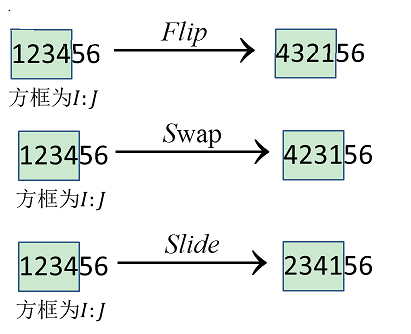希望能够对读者的理解略尽绵薄之力

代码结果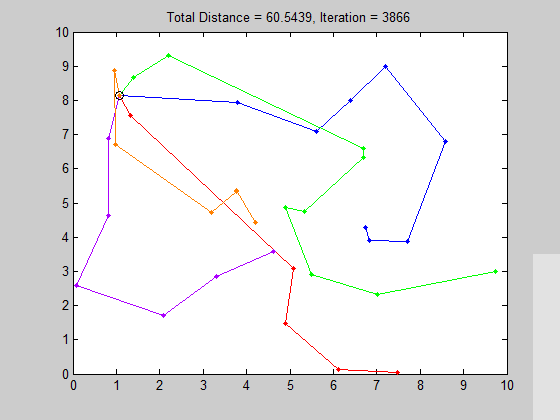统计结果：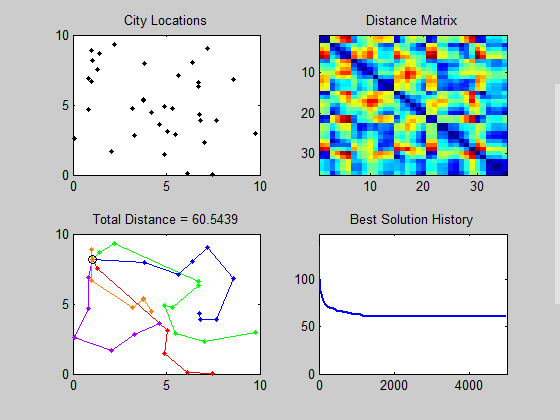关于其他MTSP问题
同一起点同一终点：
https://blog.csdn.net/weixin_42141390/article/details/103783581#%E5%9F%BA%E6%9C%AC%E7%AE%97%E6%B3%95%EF%BC%9A
随机起点随机终点
https://blog.csdn.net/weixin_42141390/article/details/103640085
展开全文遗传算法 matlab
•     基于蚁群算法的MTSP问题 蚁群算法 一、简介   20世纪50年代中期创立了仿生学，人们从生物进化的机理中受到启发。提出许多用以解决复杂优化问题的新方法，如进化规划、进化策略、遗传算法等，这些算法成功...
基于蚁群算法的MTSP问题
蚁群算法
一、简介
20世纪50年代中期创立了仿生学，人们从生物进化的机理中受到启发。提出许多用以解决复杂优化问题的新方法，如进化规划、进化策略、遗传算法等，这些算法成功地解决了一些实际问题。而同时一种对自然界蚂蚁的寻径方式进行模拟而得出的仿生算法——蚁群算法也应运而生。
二、思想与简易模型
现考察如下图所示的简单情况：我们可以清晰的看到，在蚁穴和食物之间存在两条路径，其中上路径较短，而下路径较长。在对蚂蚁的实际考察中，发现蚂蚁总会以一条最优的路径在蚁穴与食物之间进行运输，而蚂蚁又是如何判断出最优路径的呢？   研究发现，蚂蚁在寻找路径时会存在着释放信息素和受信息素导引的现象。所谓释放信息素是指蚂蚁在一条路径上行走时，会在该路径上释放信息素来指引其他蚂蚁的行径;而受信息素导引是指，蚂蚁在走过路口时，选择信息素浓度较高的路径概率会相对较大，下面我会以最简单的情况描述为什么短路径上的信息素浓度较大。假设： 1.初始时，蚂蚁从A点出发，以等概率选择路径ABD或ACD到达食物点D，然后原路返回。 2.每只蚂蚁的行走速率相同，即每单位时间行走一步。 3.假设ABD路径距离为8步，ACD的路径距离为16步。 4.假设蚂蚁每走一步释放一个信息素单位，且不考虑信息素随时间的流失。
由假设1，我们可以做出简单等效，初始时，每条路径上派一只蚂蚁。（蚂蚁数据量较大时，数量分布近似概率分布，我们可以进行归一化，假设初始每条路径只有一只蚂蚁）。上图显示的是第8个时间单位的情况，可以看到，选择路径ABD的蚂蚁已经达到食物源，而选择路径ACD的蚂蚁到达路径的中点C。此时的信息素分布如下图所示：经过16个单位时间后，选择路径ABD的蚂蚁已往返了一趟，而选择ACD的蚂蚁刚刚到达食物源D。此时信息素分布如下图所示：经过32个时间单位后，选择路径ABD与路径ACD的蚂蚁都已经回到了蚁穴，此时选择路径ABD的蚂蚁已经往返了两趟，而选择路径ACD的蚂蚁往返了一趟，此时信息素分布如下：寻找食物的过程继续，此时两只蚂蚁已经取得了食物而且回到了蚁穴，但此时两条路线上信息素分布产生了差异，由上图可知ABD路线上每一处的信息素数量与ACD路线上每一处的信息素数量之比为2:1。这意味着蚂蚁选择ABD路径的概率大于选择ACD路径概率，我们姑且假设选择路径概率与信息素浓度呈线性关系，则蚂蚁选择ABD路径的概率与选择ACD路径概率之比为2:1。   等效的做法是，我们在ABD路径上增派一只蚂蚁，则在第64个时间单位时的信息素分布如下：由上图可见，第64个时间单位时，ABD路线上每一处的信息素数量与ACD路线上每一处的信息素数量之比为3:1。假设我们将此过程无线进行下去，则按照信息素的指导，两条路径上信息素浓度之比将越来越大，且变化越来越快，形成一种正反馈，最终将导致所有的蚂蚁都会放弃ACD路线，而都选择ABD路线。
三、TSP问题的算法思路
参考链接： 蚁群算法(含matlab代码) 蚁群算法 matlab—基于蚁群算法求解Tsp旅行商问题   运用蚁群算法求解TSP问题时的基本原理是：将m个蚂蚁随机地放在多个城市，让这些蚂蚁从所在的城市出发，n步（一个蚂蚁从一个城市到另外一个城市为1步）之后返回到出发的城市。如果m个蚂蚁所走出的m条路经对应的中最短者不是TSP问题的最短路程，则重复这一过程，直至寻找到满意的TSP问题的最短路径为止。为了说明这一个算法下面用一个算法流程图来表示一下：（一）算法的相关参数  在算法中用到的变量比较多，而每个变量的对算法最终收敛的结果影响程度也不一样，在这里把一些重要的参数说明一下。

τ

i

j

\tau _{ij}

：城市i和城市j之间边

e

i

j

e_{ij}

上信息素的残留强度

Δ

τ

i

j

\Delta\tau _{ij}

：一次循环后边

e

i

j

e_{ij}

上信息素的增量

Δ

τ

i

j

k

\Delta \tau_{ij}^{k}

：一次循环之后蚂蚁k对边

e

i

j

e_{ij}

上信息素的贡献量

η

i

j

\eta _{ij}

：城市i，j之间的能见度，反映了由城市i转移到城市j的启发程度

d

i

j

d_{ij}

：城市i到城市j之间的距离

p

i

j

k

p_{ij}^{k}

：蚂蚁k从当前所在的城市到下一个城市去的概率

t

a

b

u

(

k

)

tabu(k)

：禁忌表，用于存放第k只蚂蚁已经走过的城市

Q

Q

：信息素总量，信息素总信息量为蚂蚁循环一周后向经过路径释放信息素的总量

ρ

\rho

：信息素残留系数，由于蚂蚁释放的信息量回随着时间的转移而逐渐挥发，以至于路径上的信息素不能无限递增，该系数太小时会降低算法的全局搜素能力，过大时容易使算法陷入局部最优，影响全局搜素能力。

m

m

：蚂蚁总数，在TSP问题中，每次循环当中，每只蚂蚁所走出的每条路径为TSP问题的候选解，m只蚂蚁一次循环所走出来的m条路经为TSP问题的一个解子集，所以这个解子集越大则算法的全局搜索能力越强，但是过大会使算法的收敛速度降低。如果m太小的话，算法也很容易就陷入局部最优，过早的出现停滞现象，所以选择合理的蚂蚁总数是非常重要的。

α

\alpha

：信息启发因子，反映了蚂蚁在从城市i向城市j移动时，这两个城市之间道路上所累积的信息素在指导蚂蚁选择城市j的程度，即蚁群在路径搜素中随即性因素作用的强度。

β

\beta

：期望值启发式因子，反映了蚂蚁在从城市i向城市j转移时候期望值

η

i

j

\eta _{ij}

在指导蚁群搜素中的相对重要程度。其大小反映了蚁群在道路搜素中的先验性、确定性等因素的强弱，

α

\alpha

、

β

\beta

的大小也会影响算法的收敛性。
(二) 算法过程中的关键步骤  1、上面讲了蚂蚁在选择下一个要转移的城市时候是基于概率选择的，当然这个概率不是随机概率，而是其中的一些参数来决定。假定在t时刻，蚂蚁从目前所在的i城市要选择转移去的下一个j城市，概率大小为

p

i

j

k

p_{ij}^{k}

：

p

i

j

k

(

t

)

=

{

τ

i

j

k

η

i

j

β

∑

s

∈

a

l

l

o

w

e

d

k

τ

i

s

k

(

t

)

η

i

s

β

(

t

)

j

∈

a

l

l

o

w

e

d

k

0

o

t

h

e

r

w

i

s

e

p_{ij}^{k}(t)=\left\{\begin{matrix} & \frac{\tau_{ij}^{k}\eta _{ij}^{\beta }}{\sum_{s\in allowed_{k}}\tau_{is}^{k}(t)\eta_{is}^{\beta}(t)} &j\in allowed_{k} \\ \\ &0 &otherwise \end{matrix}\right.

其中

a

l

l

o

w

e

d

k

allowed_{k}

表示允许蚂蚁k下一步可容许去的城市的集合，

τ

i

j

α

\tau_{ij}^{\alpha}

为边

e

i

j

e_{ij}

上的信息素因数，

η

i

j

β

\eta_{ij}^{\beta}

为城市i，j间能见度因数。至于这个过程具体是怎么实现的，在程序中会有相关的源码。
2、对任意两个城市i，j之间道路对应的边

e

i

j

e_{ij}

信息素增量按照下式进行：

{

τ

i

j

(

t

+

1

)

=

ρ

τ

i

j

(

t

)

+

Δ

τ

i

j

(

t

,

t

+

1

)

Δ

τ

i

j

(

t

,

t

+

1

)

=

Σ

k

=

1

m

Δ

τ

i

j

k

(

t

,

t

+

1

)

\left\{\begin{matrix} &\tau_{ij}(t+1)=\rho\tau_{ij}(t)+\Delta \tau_{ij}(t,t+1)\\ \\&\Delta\tau_{ij}(t,t+1)=\Sigma_{k=1}^{m}\Delta\tau_{ij}^{k}(t,t+1) \end{matrix}\right.

其中，

Δ

τ

i

j

k

(

t

,

t

+

1

)

\Delta\tau_{ij}^{k}(t,t+1)

为蚂蚁k对边

e

i

j

e_{ij}

上所贡献的信息素增量，

Δ

τ

i

j

(

t

,

t

+

1

)

\Delta\tau_{ij}(t,t+1)

是经过边

e

i

j

e_{ij}

的所有蚂蚁对边

e

i

j

e_{ij}

的信息素量贡献，

ρ

\rho

为信息素残留系数。对于

Δ

τ

i

j

k

(

t

,

t

+

1

)

\Delta\tau_{ij}^{k}(t,t+1)

的调整方式不同，可以将蚁群算法分为三种模型：蚁密模型、蚁量模型和蚁周模型。
2.1 蚁密模型   在蚁密模型当中，每一只蚂蚁在经过城市i,j时，对该边

e

i

j

e_{ij}

所贡献的信息素增量为常量，每个单位长度是Q：2.2 蚁量模型   在蚁量模型中，一只蚂蚁k在经过城市i,j之间边

e

i

j

e_{ij}

时，对该边所贡献的信息素增量为变量，即每单位长度上为Q/

d

i

j

d_{ij}

，它与城市i,j之间的路径长度

d

i

j

d_{ij}

有关，具体为：2.3 蚁周模型   上述两种模型，对两城市之间边

e

i

j

e_{ij}

上信息素贡献的增量在蚂蚁k经过边的同时完成，而蚁周模型对边

e

i

j

e_{ij}

信息素的增量是在本次循环结束时才进行更新调整。一只蚂蚁在经过城市i，j时对边

e

i

j

e_{ij}

上贡献的增量为每单位长度

Q

/

L

k

Q/L_{k}

，

L

k

L_{k}

为蚂蚁在本次循环走出路径的长度。本文章就是基于蚁周模型来实现的，介绍完上述的几个关键步骤以后，就要用matlab来实现MTSP问题了，另外，关于TSP文体的算法主体框架可参考上述链接中的三篇文章，本文不再详述。
四、MTSP算法实现
本文的重点是运用蚁群算法解决MTSP问题，而MTSP问题的算法框架与TSP问题的算法框架基本相似，但关于蚂蚁单位的处理有一些需要注意的细节，首先让我们考察一个具体的问题。附件中列出了陕西曲线公路里程表，现在有两个旅行者从碑林区出发，求合计拜访每个区县一次且仅一次且最后都回到碑林区的最短路径。   和单纯的TSP问题不同的是，我们的单位不再是一只蚂蚁，而是一对蚂蚁，我们通过一对蚂蚁进行全局搜索。这里便涉及到几个TSP问题不需要考虑到的问题，   问题一：如何让两只蚂蚁协同搜索路径，即每进行一个时间单位，应该是哪一只蚂蚁进行搜索，亦或是两只蚂蚁一起搜索。   问题二：两只蚂蚁释放的信息素是否为同一种，即确定下一步搜索路径时，是要将两种蚂蚁释放的信息素综合起来考虑，还是应该分开考虑两种信息素的影响. 解决方法：   1.若每次让两只蚂蚁一起搜素，则每只蚂蚁会固定的走访一半的城市，而往往最优的路径并不是每只蚂蚁一定要走访一半的城市。所以我们选择对两只蚂蚁即将走访的两条路径，选择路径较短的蚂蚁进行下一步的路径搜索。   2.经过试验发现，信息素选择为一种与两种的实验结果影响不大，故将两种蚂蚁释放的信息素综合起来考虑。
多旅行商问题的代码如下所示:  (具体的实现细节详见代码注释)
D = P3;
n = 107;
m = 50;                              % 蚂蚁对数，每对蚂蚁分为蚂蚁1与蚂蚁2
alpha = 1;                           % 信息素重要程度因子
beta = 5;                            % 启发函数重要程度因子
rho = 0.1;                           % 信息素挥发因子
Q = 1;                               % 常系数（信息素释放量）
Eta = 1./D;                          % 启发函数
Tau = ones(n,n);                     % 信息素矩阵
Table1 = zeros(m,n);                 % 蚂蚁1路径记录表
Table2 = zeros(m,n);                 % 蚂蚁2路径记录表
iter = 1;                            % 迭代次数初值
iter_max = 150;                      % 最大迭代次数
Route_best1 = zeros(iter_max,n);     % 蚂蚁1各代最佳路径
Route_best2 = zeros(iter_max,n);     % 蚂蚁2各代最佳路径
Length_best = zeros(iter_max,1);     % 各代最佳路径的长度
Length_ave  = zeros(iter_max,1);     % 各代路径的平均长度

while iter <= iter_max
start = ones(m,1)+1;           %初始出发城市为2城市，即碑林区
Table1(:,1) = start;
Table2(:,1) = start;
citys_index = 1:n;

% 逐个蚂蚁路径选择
for i = 1:m
% 逐个城市路径选择
for j = 2:n
tabu1 = Table1(i,1:(j - 1));       %蚂蚁1已访问的城市集合
tabu1(tabu1==0) = [];              %把表中的0元素舍去，因为无意义
tabu2 = Table2(i,1:(j - 1));       %蚂蚁2已访问的城市集合
tabu2(tabu2==0) = [];              %把表中的0元素舍去，因为无意义
tabu = [tabu1 tabu2];
allow_index = ~ismember(citys_index,tabu);
allow = citys_index(allow_index);       %待访问的城市集合
P1 = allow;
P2 = allow;
% 计算城市间转移概率
for k = 1:length(allow)
P1(k) = Tau(tabu1(end),allow(k))^alpha * Eta(tabu1(end),allow(k))^beta;
P2(k) = Tau(tabu2(end),allow(k))^alpha * Eta(tabu2(end),allow(k))^beta;
end
P1 = P1/sum(P1);
P2 = P2/sum(P2);
% 轮盘赌法选择下一个访问城市
Pc1 = cumsum(P1);
Pc2 = cumsum(P2);
target_index1 = find(Pc1 >= rand);
target_index2 = find(Pc2 >= rand);
target1 = allow(target_index1(1));
target2 = allow(target_index2(1));
% 计算蚂蚁1与蚂蚁2到下一个城市的路径长度，选择路径长度较少的蚂蚁进行行走
% 假设蚂蚁1行走，则蚂蚁1的路径表将添加下一个城市的标号，而蚂蚁2的路径表将添加0
lengthant1 = D(tabu1(end), target1);
lengthant2 = D(tabu2(end), target2);
if( lengthant1 <= lengthant2)
Table1(i,j) = target1;
else
Table2(i,j) = target2;
end
end
end

% 计算各个蚂蚁对的路径距离
Length1 = zeros(m,1);    % 蚂蚁1路径距离之和
Length2 = zeros(m,1);    % 蚂蚁2路径距离之和
for i = 1:m
Route1 = Table1(i,:);
Route1(Route1 == 0) = [];    %舍去表中0元素
Route2 = Table2(i,:);
Route2(Route2 == 0) = [];    %舍去表中0元素
for j = 1:length(Route1)-1
Length1(i) = Length1(i) + D(Route1(j),Route1(j + 1));
end
Length1(i) = Length1(i) + D(Route1(end),Route1(1));
for j = 1:length(Route2)-1
Length2(i) = Length2(i) + D(Route2(j),Route2(j + 1));
end
Length2(i) = Length2(i) + D(Route2(end),Route2(1));
end
Length = Length1 +  Length2;
% 计算最短路径距离及平均距离
if iter == 1
[min_Length,min_index] = min(Length);
Length_best(iter) = min_Length;
Length_ave(iter) = mean(Length);
Route_best1(iter,:) =  Table1(min_index,:);
Route_best2(iter,:) =  Table2(min_index,:);
else
[min_Length,min_index] = min(Length);
Length_best(iter) = min(Length_best(iter - 1),min_Length);
Length_ave(iter) = mean(Length);
if Length_best(iter) == min_Length
Route_best1(iter,:) =  Table1(min_index,:);
Route_best2(iter,:) =  Table2(min_index,:);
else
Route_best1(iter,:) = Route_best1((iter-1),:);
Route_best2(iter,:) = Route_best2((iter-1),:);
end
end
% 更新信息素
Delta_Tau = zeros(n,n);
% 逐个蚂蚁计算
for i = 1:m
Route1 = Table1(i,:);
Route1(Route1 == 0) = [];     %舍去表中0元素
Route2 = Table2(i,:);
Route2(Route2 == 0) = [];     %舍去表中0元素
% 逐个城市计算
for j = 1:length(Route1)-1
Delta_Tau(Route1(j),Route1(j+1)) = Delta_Tau(Route1(j),Route1(j+1)) + Q/Length1(i);
end
for j = 1:length(Route2)-1
Delta_Tau(Route2(j),Route2(j+1)) = Delta_Tau(Route2(j),Route2(j+1)) + Q/Length2(i);
end
Delta_Tau(Route1(end),Route1(1)) = Delta_Tau(Route1(end),Route1(1)) + Q/Length1(i);
Delta_Tau(Route2(end),Route1(1)) = Delta_Tau(Route2(end),Route1(1)) + Q/Length2(i);
end
Tau = (1-rho) * Tau + Delta_Tau;
% 迭代次数加1，清空路径记录表
iter = iter + 1;
Table1 = zeros(m,n);
Table2 = zeros(m,n);
end

[Shortest_Length,index] = min(Length_best);
Shortest_Route1 = Route_best1(index,:);
Shortest_Route1(Shortest_Route1 == 0) = [];
Shortest_Route2 = Route_best2(index,:);
Shortest_Route2(Shortest_Route2 == 0) = [];
disp(['最短距离: ' num2str(Shortest_Length)]);
disp(['最短路径1: ' num2str([Shortest_Route1 Shortest_Route1(1)])]);
disp(['最短路径2: ' num2str([Shortest_Route2 Shortest_Route2(1)])]);
figure(2)
plot(1:iter_max,Length_best,'b',1:iter_max,Length_ave,'r:')
legend('最短距离','平均距离')
xlabel('迭代次数')
ylabel('距离')
title('各代最短距离与平均距离对比')
% r = TSP;
% sum = 0;
% for  i = 1:107
%     sum = sum+D(r(i),r(i+1));
% end
% sum

实验结果：最短距离: 7192.7
最短路径1: 2    6    9   12   35   34   36   37   39   40   51    7   16   14   15   17   66   63   62   61   54   58   57   59   60   83   82   81   78   79   80   89   84   85   86   87   88   56   55   64   65   50   49   48   47   52   46   53   45   44   43   10  100  102  103  101  104  105  106   93   92   91   90   98   99   96   97   95   94   75   71   69   70   68   67   72   73   74   76   28   77    2
最短路径2: 2    3    5    1    4    8   13   32   33   31   30  107   42   41   11   23   22   21   20   18   19   26   25   29   24   27   38    2
>>

陕西公路里程表数据 提取码：1234 才疏学浅，欢迎大家指正~
展开全文算法 matlab 人工智能
• 4.2 MTSP问题python实现 对于该MTSP问题，笔者没有找到相关的python实现包，因此只能手撸代码如下： import numpy as np from matplotlib import pyplot as plt import math import copy import random def init_...
写在前面
遗传算法是一种求解NPC问题的启发式算法，属于仿生进化算法族的一员。仿生进化算法是受生物行为启发而发明的智能优化算法，往往是人们发现某种生物的个体虽然行为较为简单，但生物集群通过某种原理却能表现出智能行为。于是不同的人研究不同的生物行为原理，受到启发而发明出新的仿生进化算法。比如免疫优化算法，蚁群算法，模拟退火算法等，这些算法以后也会简单介绍。 本文的主题是遗传算法，该算法也是受到生物行为启发。物竞天择，适者生存，优胜劣汰，是该优化算法的核心思想。笔者在业务中需要用到遗传算法求解TSP问题，但是网上能查找到的资料对遗传算法的讲解不够通俗易懂，往往上来就是遗传变异交叉，对于我这样的初学者来说有点不知所云，于是不得不直接看源码，一行一行地理解代码的意思，才弄懂了原理。这种方法对于初学者和编程基础薄弱者颇为困难，而且费时费力，苦不堪言。同时，由于读者可能熟练掌握的是不同的语言，因此若代码是某一种语言编写的，那么掌握其他语言的读者很可能难以吸收，浪费了资源。此外，网上关于TSP问题的资料很多，但是关于MTSP问题的资料却凤毛麟角。因此有了创作本文的意图，旨在用最通俗详尽的语言深入浅出地解释遗传算法解TSP、MTSP问题的原理及应用
遗传算法解TSP问题原理
一、TSP问题
旅行商问题，即TSP问题（Traveling Salesman Problem）又译为旅行推销员问题、货郎担问题，是数学领域中著名问题之一。假设有一个旅行商人要拜访n个城市，他必须选择所要走的路径，路径的限制是每个城市只能拜访一次，而且最后要回到原来出发的城市。路径的选择目标是要求得的路径路程为所有路径之中的最小值。 想要求解出TSP问题的最优解，目前唯一的方法是穷举出所有的路径。然而，路径的数量级是n!，也就是目标点数量的阶乘。当n为14时，n!已经大于800亿。当n更大，为30，40 时，更是天文数字，即使计算机一秒钟计算一亿次，其求解时间也远大于我们的寿命。因此，穷举法解TSP问题根本不可行，需要别的启发式算法来代替。
二、遗传算法解TSP原理
2.1
笔者认为，大多数的遗传算法讲解让人难以理解的原因在于其术语名称的意义不明确，往往与其实际意义相去甚远。譬如，什么是适应度函数，什么是遗传，变异，交叉。乍一听总有不知所云的感觉，这跟TSP问题求最短路径有什么关系。因此本文将从TSP问题出发，讲解遗传算法的思想和原理。
2.2
对于TSP问题，我们拿到的是n个目标点的坐标，作为例子，我们视为面对的是n=10个城市。有了坐标，我们便可以计算出n个城市之间的距离，得到一个n*n的矩阵，这个矩阵表示的是城市之间两两连接形成的无向图，边的权重是城市之间的距离，而TSP问题则是从图中找出一个包含所有城市的最小的环。
2.3
问题明确了，接下来就是遗传算法登场了。
2.3.1 种群初始化
首先是初始化种群，TSP里的初始化种群其实就是一个长度为n=10的包含1~n(这个例子里为10)且不含重复元素的序列，其意义就是一个人从某个点出发，随机访问下一个未访问过的城市，直到所有的城市都访问完毕，他再从最后一个城市返回出发城市。他的轨迹就是一个包含了所有城市且没有重复访问的环。种群数量设为M，那么该种群初始化的意义就是M个人独立、随机、不重复地访问一遍各个城市，单个个体轨迹构成一个环。
2.3.2 适应度计算
M个个体的轨迹得到了，这些轨迹是随机游走得到的，当然很可能远远不包含属于最优解，而是比最优解坏得多。但是，随机游走的路径也有大有小，有好有坏。因此需要有一个函数衡量个体的好坏，也就是环路径的长短。 那么这个函数就被称为适应度函数，其功能是衡量个体的好坏。对于TSP问题，其适应度函数当然是距离相关的了。个体的好坏，衡量标准就是该个体序列的路径长度。路径越长，个体越“坏”，路径越短，个体越“好”。因此，设序列为x,那么该序列的路径长度便是d(x)，而适应度函数则应该取为1/d(x)，适应度越大，个体越优。
2.3.3 选择
有了每个个体的适应度，就能评价每个个体的好坏。物竞天择，优胜劣汰，将优秀的个体选择出来进行交配，以期得到更好的个体，并由此不断进化，一代代传承，后代不断比前一代变得更好，最终收敛，种群中的某个个体达到了优秀的极限，便是最优解。 对于TSP问题，选择的具体操作是，计算出所有个体的适应度，也就是路径距离的倒数。然后将所有个体的适应度归一化，得到概率。然后从数量为n的个体中以轮盘赌的形式选择出若干个个体，视为优秀个体。 举个例子，为了简单起见，设种群大小m=4。并计算出了四个个体的适应度分别为1，2，3，4。对适应度进行归一化得到概率：0.1，0.2，0.3，0.4。那么这四个概率就可以构成一个轮盘，每个个体对应的被选择的概率分别为0.1，0.2，0.3，0.4。随后，在这个转盘上转动m次，此处为4。将选中的个体放入一个集合，未选中的个体抛弃。 注意，这是一种重复抽样的选择方式，并且重复抽到的个体不去重。每次抽样，轮盘都是相同的轮盘，并没有改变。比如，在这个例子中，种群数量为4，那么就要抽取四次，那么0.4对应的个体很可能被多次抽样，而0.1对应的个体很可能未被抽到，直接淘汰。那么被选择的个体集合就很可能含有多个适应度为4的个体，并且被选择的集合的种群数量仍旧为m，只不过抛弃了较坏的个体，而可能保留了多个优秀的个体。个体越优秀，被重复选择的概率就越大。
2.3.4 交叉（遗传）
遗传算法中的交叉属于遗传算法的核心以及关键步骤，其思想受启发于生物遗传中的染色体交叉。 对于TSP问题，有如下的交叉方式进行交叉。
Partial-Mapped Crossover（部分映射交叉）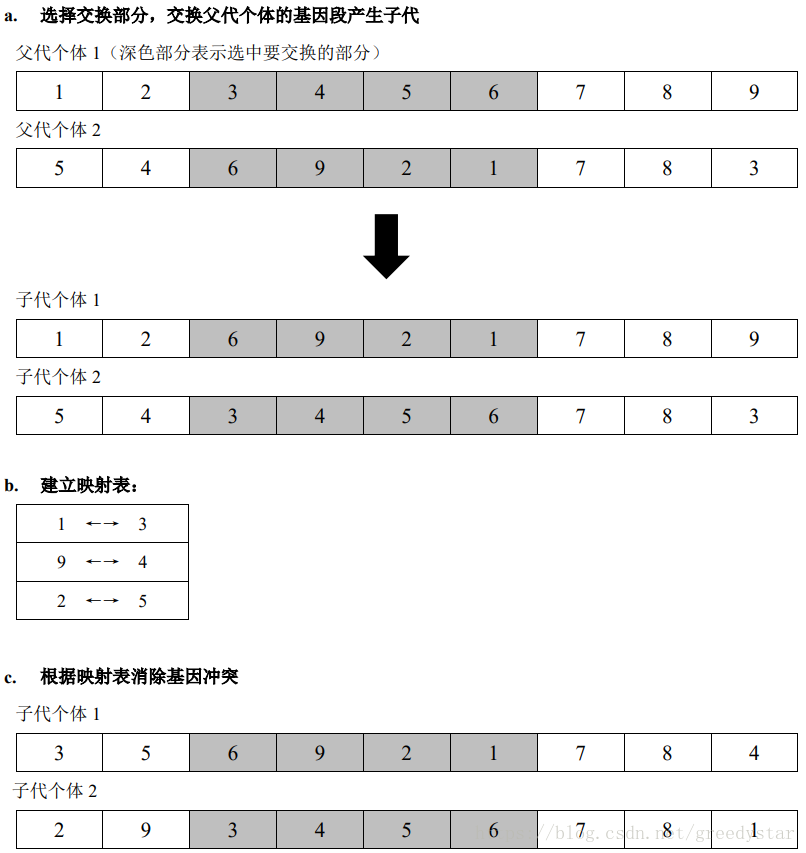Order Crossover（顺序交叉）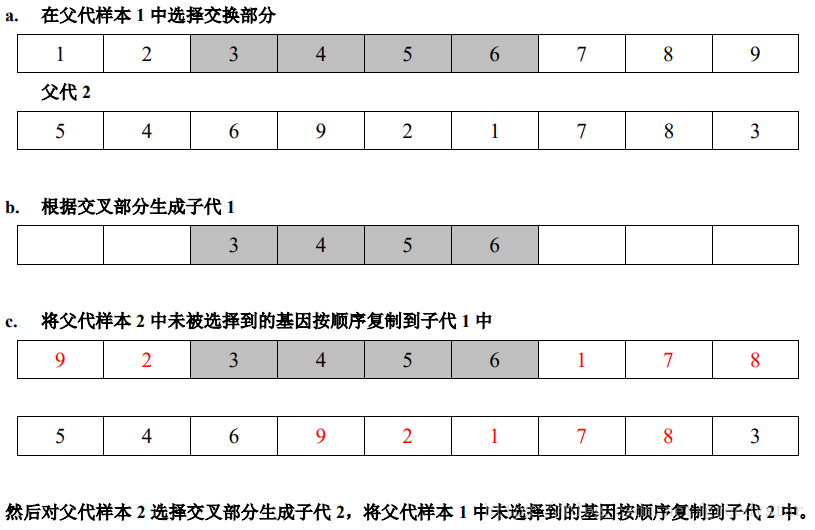Position-based Crossover（基于位置的交叉）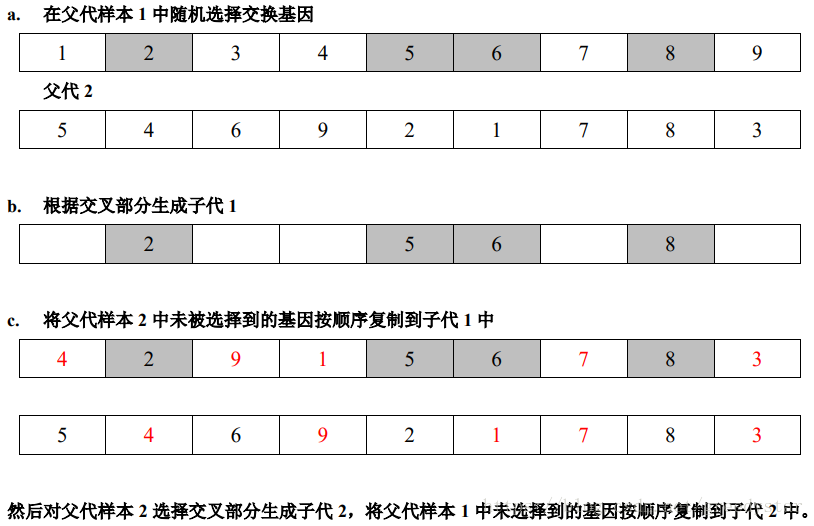那么，在这个被选择的大小为种群数量m的新的种群中，如何通过上述方式交叉呢？这里有一个超参数pc(probability of crossover)。将种群打乱之后，随机两两组合。每对组合以pc的概率采用上述方式之一进行交叉，以（1-pc）的概率不进行交叉，而是二者直接进入下一代。pc的值一般在0.5到0.9之间。 通过上述方式，便能产生一个同样大小为m的新的种群。此刻，选择、交叉的过程已经完成，还剩最后一步操作——变异。
2.3.5 变异
对于上一步交叉得到的大小为m的种群，以pm的概率选择出部分个体进行变异操作。选择出来的个体序列随机选择两个位置上的数进行交换。如，其中一个被选择的个体的序列为196278354，那么在这个序列中随机选择两个位置上的数，比如第二个数9和第四个数2，进行交换。那么得到的变异后的序列就是：126978354，变异操作完成。
2.3.6 传承
至此，种群的迭代更新方式基本阐述完毕，还剩下最后一步操作，传承。这是说，将上一轮中最优的个体（对于TSP问题，也就是路径距离最短的序列），保留下来，并用他替换掉新产生的种群中最差的个体。这一步的意义在于，上一轮中最优的个体被选择的几率也最大，但是它与其他个体交叉之后得到的新个体不一定优于它自己。如果这样，那么这个最优的个体便被覆盖、迭代掉了，这样很可能造成下一代中的所有个体都比上一代的最优个体差，优秀的基因被淘汰。为了避免这样的情况，需要这一步传承，保证每次迭代之后的最优个体不坏于之前出现的所有个体。
三、遗传算法解TSP问题python实现
对于单纯的TSP问题，用python求解非常简便，原因在于python已有十分强大的遗传算法工具包，用户只需要将需要求解的目标点位置转化成n*n的距离矩阵distance_matrix，然后直接调用python里的库sko.GA即可，下面是示例代码。读者不用担心直接调包无法了解算法实现的细节，在下一节的MTSP问题本文将基于纯python手写实现遗传算法。
// An highlighted block
def cal_total_distance(routine):
cal_total_distance(np.arange(num_points))
'''
num_points, = routine.shape
return sum([distance_matrix[routine[i % num_points], routine[(i + 1) % num_points]] for i in range(num_points)])
from sko.GA import GA_TSP
#num_points为城市数量，size_pop为种群数量，max_iter为迭代次数
#prob_mut为变异率
ga_tsp = GA_TSP(func=cal_total_distance, n_dim=num_points, size_pop=50, max_iter=2000, prob_mut=1)
best_points, best_distance = ga_tsp.run()

其中best_points返回的是最优路径的顺序，best_distance返回的是最优路径的距离。
用python工具包实现TSP问题就是这样简洁容易。在下一节，要讨论的是更为复杂的MTSP问题。
四、遗传算法解MTSP问题
4.1问题描述
问题描述：m个旅行商去旅游 n个城市，一种规定是都必须从同一个出发点出发，而且返回原出发点，需要将所有的城市遍历完毕，每个城市只能游历一次，但是为了路径最短可以路过这个城市多次。这个就是多旅行商问题。是在TSP问题的基础上进行了扩展。
4.2 问题分析
关于MTSP问题，可以找到的资料比TSP要少很多。对于该问题的求解，可以基于TSP问题的思路进行修改。首先需要明确具体的业务需求，是多个旅行商从不同的城市出发，遍历所有的目标点并回到自己的原点，还是都从同一个点出发回到所有起点，还是回到同一个点但该点不是起点。另外，每个旅行商访问城市的数量是任意的，还是两个旅行商需要访问的城市数目是大致相等的，还是规定了每个旅行商访问的数量具体是多少？ 不论问题是哪种，其思想是差不多的。本文以多个旅行商从同一点出发，回到同一个起点，且每人访问的城市数量相同的情形。首先，跟TSP问题一样，初始化大小为M的种群，每个个体是一个不含重复点的长度为n的序列。然后重点是设置断点，将该序列拆分成m段，也就是旅行商的数量。此后，将m段序列的首尾都添上起点，形成m个旅行商各自的环路径，然后计算各个环的距离的总和的倒数，作为适应度函数的值，后续操作按照TSP问题的遗传算法求解即可。在本例中，考虑的m取2，两位旅行商。断点取序列的中间，也就是序列平均分为两半。若读者想不限制各位旅行商的访问城市的数目相等，只需要设置断点在不同的位置即可。
4.2 MTSP问题python实现
对于该MTSP问题，笔者没有找到相关的python实现包，因此只能手撸代码如下：
import numpy as np
from matplotlib import pyplot as plt
import math
import copy
import random
def init_population(length, num):
li = list(range(length))
print(li)
population = []
for i in range(num):
random.shuffle(li)
population.append(copy.deepcopy(li))
return population
def aimFunction(entity, DMAT, break_points):
"""
目标函数
:param entity: 个体
:param DMAT: 距离矩阵
:param break_points: 切断点
:return:适应度函数值
"""
distance = 0
break_points.insert(0, 0)
break_points.append(len(entity))
routes = []
for i in range(len(break_points) - 1):
routes.append(entity[break_points[i]:break_points[i + 1]])
# print(routes)
#上面代码的作用是将路径拆成m段。break_points的长度设置为k时，则将路径拆分成了k+1段，此处的k 取的1。
for route in routes:
if 0 in route:
route.remove(0)
route.insert(0,0)
route.append(0)#此处给每段路径添上首尾点0，因为本例所有旅行商都从0这个城市出发，具体从哪里出发根据实际问题修改该设定。
for i in range(len(route)-1):
distance += DMAT[route[i],route[i+1]]

return 1.0/distance
#返回种群所有个体的适应度列表
def fitness(population, DMAT, break_points, aimFunction):
"""
适应度
:param population: 种群
:param DMAT: 距离矩阵
:param break_points:切断点
:param aimFunction: 目标函数
:return:
"""

value = []
for i in range(len(population)):
value.append(aimFunction(population[i], DMAT, copy.deepcopy(break_points)))
# weed out negative value
if value[i] < 0:
value[i] = 0
return value
#这里传入的是种群和每个个体的适应度
#这里的轮盘赌与蚁群算法的有一定区别。这里对适应度归一化得到概率之后，每个个体被选中的概率就是这个概率
#对每次被选中的个体的数目没有限制，完全随机，限制的是选择次数n与种群数目相同，使得新的种群数量与旧的种群一致
def selection(population, value):
# 轮盘赌选择
fitness_sum = []
for i in range(len(value)):
if i == 0:
fitness_sum.append(value[i])
else:
fitness_sum.append(fitness_sum[i - 1] + value[i])

for i in range(len(fitness_sum)):
fitness_sum[i] /= sum(value)

# select new population
population_new = []
for i in range(len(value)):
rand = np.random.uniform(0, 1)
for j in range(len(value)):
if j == 0:
if 0 < rand and rand <= fitness_sum[j]:
population_new.append(population[j])

else:
if fitness_sum[j - 1] < rand and rand <= fitness_sum[j]:
population_new.append(population[j])
return population_new
#对于被选中的双亲，随机两两组合。并以pc的概率交配
#若没有被选择交配，则直接双亲进入下一代。如果被选择，则交换中间片段。
def amend(entity, low, high):
"""
修正个体
:param entity: 个体
:param low: 交叉点最低处
:param high: 交叉点最高处
:return:
"""
length = len(entity)
cross_gene = entity[low:high]  # 交叉基因
not_in_cross = []  # 应交叉基因
raw = entity[0:low] + entity[high:]  # 非交叉基因

for i in range(length):
if not i in cross_gene:
not_in_cross.append(i)

error_index = []
for i in range(len(raw)):
if raw[i] in not_in_cross:
not_in_cross.remove(raw[i])
else:
error_index.append(i)
for i in range(len(error_index)):
raw[error_index[i]] = not_in_cross[i]

entity = raw[0:low] + cross_gene + raw[low:]

return entity

def crossover(population_new, pc):
"""
交叉
:param population_new: 种群
:param pc: 交叉概率
:return:
"""
half = int(len(population_new) / 2)
father = population_new[:half]
mother = population_new[half:]
np.random.shuffle(father)
np.random.shuffle(mother)
offspring = []
for i in range(half):
if np.random.uniform(0, 1) <= pc:
# cut1 = np.random.randint(0, len(population_new))
# if cut1 >len(father[i]) -5:
#     cut2 = cut1-5
# else:
#     cut2 = cut1+5
cut1 = 0
cut2 = np.random.randint(0, len(population_new))
if cut1 > cut2:
cut1, cut2 = cut2, cut1
if cut1 == cut2:
son = father[i]
daughter = mother[i]
else:
son = father[i][0:cut1] + mother[i][cut1:cut2] + father[i][cut2:]
son = amend(son, cut1, cut2)
daughter = mother[i][0:cut1] + father[i][cut1:cut2] + mother[i][cut2:]
daughter = amend(daughter, cut1, cut2)

else:
son = father[i]
daughter = mother[i]
offspring.append(son)
offspring.append(daughter)

return offspring
#这里的变异是最简单的变异法则，直接随机选取两个位置上的数进行交换
def mutation(offspring, pm):
for i in range(len(offspring)):
if np.random.uniform(0, 1) <= pm:
position1 = np.random.randint(0, len(offspring[i]))
position2 = np.random.randint(0, len(offspring[i]))
# print(offspring[i])
offspring[i][position1],offspring[i][position2] = offspring[i][position2],offspring[i][position1]
# print(offspring[i])
return offspring
#主函数
if __name__ == '__main__':

#这里的graph为距离矩阵，需要自己定义后传入

DMAT=graph
break_points = [len(graph)//2]#这里是将所有城市从中间断开成两段。读者可以根据需求切断成任意段（每段代表一个旅行商），并且可以在任意位置切断。
population = init_population(len(graph), 90)

t = []
dic_result={}
for i in range(20000):
# selection
value = fitness(population, DMAT, break_points, aimFunction)
population_new = selection(population, value)
# crossover
offspring = crossover(population_new, 0.65)
# mutation
population = mutation(offspring, 0.02)
# if i % 1 == 0:
#     show_population(population)
result = []
for j in range(len(population)):
result.append(1.0 / aimFunction(population[j], DMAT, copy.deepcopy(break_points)))

t.append(min(result))
min_entity = population[result.index(min(result))]
routes = []
break_points_plt = copy.deepcopy(break_points)
break_points_plt.insert(0, 0)
break_points_plt.append(len(min_entity))
for i in range(len(break_points_plt) - 1):
routes.append(min_entity[break_points_plt[i]:break_points_plt[i + 1]])
for route in routes:
if 0 in route:
route.remove(0)
route.insert(0,0)
route.append(0)
dic_result[min(result)]=routes
if i % 400 == 0:
min_entity = population[result.index(min(result))]
routes = []
break_points_plt = copy.deepcopy(break_points)
break_points_plt.insert(0, 0)
break_points_plt.append(len(min_entity))
for i in range(len(break_points_plt) - 1):
routes.append(min_entity[break_points_plt[i]:break_points_plt[i + 1]])
for route in routes:
if 0 in route:
route.remove(0)
route.insert(0,0)
route.append(0)
for route in routes:
print(route)
print(min(t))#每次迭代的最优路径
print('最短路径距离之和为',min(t),'米')
plt.plot(t)#画出每次迭代的最优个体对应的环路程


这里我传入的是一个22*22的距离矩阵，读者在使用时需要自定义矩阵和断点。该矩阵第一行元素如下： [0.00000e+00, 1.00000e+00, 6.83910e+04, 6.81450e+04, 4.29630e+04, 4.13760e+04, 7.52490e+04, 7.09670e+04, 7.82330e+04, 7.34120e+04, 4.08460e+04, 4.09140e+04, 8.49820e+04, 8.49350e+04, 8.18330e+04, 5.04900e+04, 4.71150e+04, 3.36550e+04, 3.35980e+04, 3.16970e+04, 6.96790e+04, 7.21160e+04, 6.93410e+04, 5.62990e+04, 5.16090e+04] 断点设置在中间，也就是22整除2，将22个点均分成两份，每份11个点，然后两位旅行商分别从第一个点出发，巡游各自需要访问的点，再回到起点。具体迭代过程在上文中已经阐述。最终得到的结果plot如下：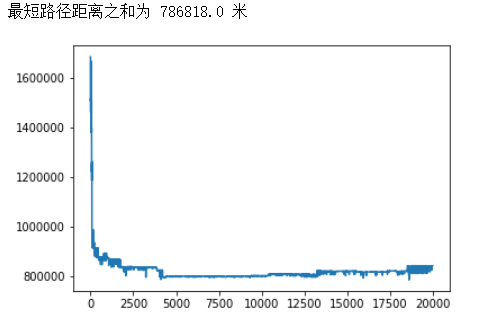五、总结
遗传算法是一种仿生进化启发式算法，该算法不保证搜索到最优解，但是借鉴生物进化中优胜劣汰，物竞天择的思想，随机初始化个体之后使用适应度函数对个体的优劣进行评测，然后选择较为优秀的个体进行交配，较劣的个体淘汰。人们期望优秀的个体之间交配能产生更优的个体，如此代代传承进化下去，得到越来越优秀的个体。当然，优秀的个体之间交叉之后，并不保证其后代一定优于双亲，因此遗传算法也可能收敛到局部最小值或者近优值。当然，这也是所有仿生进化算法的特性。目前还没有能完全解决NPC问题的有效算法。
展开全文算法 python
• 本篇文章是利用遗传算法来解决MTSP或者MVRP问题的，至于遗传算法较为详尽的介绍的话，在我上一篇文章中有介绍，这里就不再啰嗦了。 1、问题简述 在本文中，MTSP问题就是有一组旅行者需要遍历完给定的所有城市，...
本篇文章是利用遗传算法来解决MTSP或者MVRP问题的，至于遗传算法较为详尽的介绍的话，在我上一篇文章中有介绍，这里就不再啰嗦了。
1、问题简述
在本文中，MTSP问题就是有一组旅行者需要遍历完给定的所有城市，并且回到起点，要求其总体的旅行距离为最小；MVRP问题就是把一组旅行者变为一组机器人而已。在本文中所有旅行者的起点是不确定的，也就是说旅行者可以随机从一个城市出发，并且在最后要回到起点城市。假设旅行者人数为nSalesmen = k，城市位置为xy，那么整体的图会有K个回路，且K个回路中没有子回路了。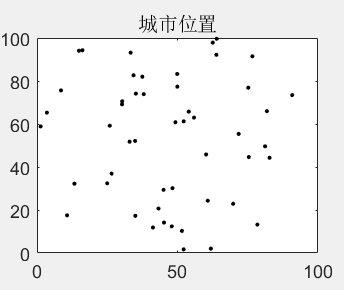城市位置分布图
2、染色体编码问题
染色体编码方式采用的仍然是十进制编码方式，与TSP问题不同的是：每一个个体都会有一个与之相对应的染色体分隔点的集合。把染色体进行分隔的目的是区别每一个旅行者的路径。如下图所示，假设有3个机器人，需要去访问14个城市，则染色体就是14个城市序号的随机排列，3个机器人则对应有两个分隔点标记，如图产生的两个分隔点标记为5和11的位置。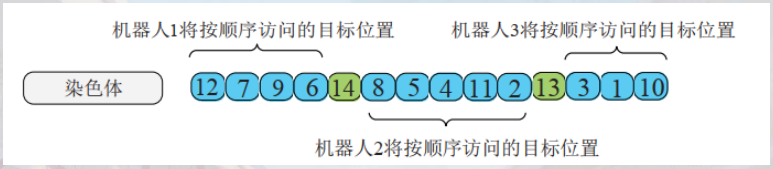染色体分隔演示
若每一个旅行者有最小旅行城市的限制的话，也就是每一个旅行者至少需要旅行minTour个城市的话，染色体分隔点会有所不同。集中在染色体前端的位置作为分隔点的概率会小很多，且分隔点与分隔点之前必须要有minTour个城市，则需要根据概率来限制分隔点的选择了。
3、遗传算法流程
重新回顾一下遗传算法的流程吧！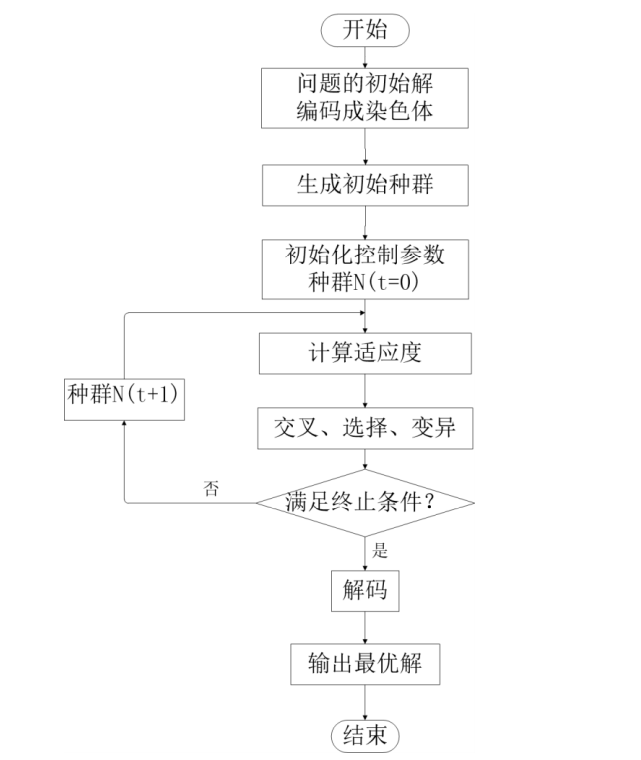GA流程
在本文中问题的初始解包括旅行者初始路线的初始化和染色体分隔点的初始化，两者是一一对应的关系。
4、Matlab代码及其结果
function varargout = mtsp_ga(xy,dmat,nSalesmen,minTour,popSize,numIter,showProg,showResult)
%若无参数输入，参数初始化
nargs = 8;
for k = nargin:nargs-1
switch k
case 0
xy = 100*rand(50,2);%随机生成50个城市坐标，rand(0~1)
case 1
N = size(xy,1);%城市个数
a = meshgrid(1:N);%生成N*N升序矩阵
dmat = reshape(sqrt(sum((xy(a,:)-xy(a',:)).^2,2)),N,N);%计算距离矩阵。装置，生成N*N的距离矩阵
case 2
nSalesmen = 5;%旅行者个数
case 3
minTour = 3;%最小旅行距离
case 4
popSize = 80;%种群个数
case 5
numIter =5e3;%迭代次数
case 6
showProg =1;%展示迭代过程
case 7
showResult = 1;%展示最终结果
otherwise
end
end
%对输入参数进行扫描
[N,dims] = size(xy);%城市个数、维数
[nr,nc] = size(dmat);%距离矩阵的行数和列数
if N~=nr||N~=nc
error('城市坐标或距离矩阵输入错误')
end
n =N;

%数据检查
nSalesmen = max(1,min(n,round(real(nSalesmen(1)))));
minTour = max(1,min(floor(n/nSalesmen),round(real(minTour(1)))));
popSize = max(8,8*ceil(popSize(1)/8));
numIter = max(1,round(real(numIter(1))));
showProg = logical(showProg(1));%将数值转变为逻辑值
showResult=logical(showResult(1));

%初始化路径分隔点(染色体分隔点)
nBreaks = nSalesmen -1;
dof = n - minTour*nSalesmen; %自由城市数目
for k = 2:nBreaks
end

%% 初始化种群
popRoute = zeros(popSize,n);%80个体
popBreak = zeros(popSize,nBreaks);%每个种群都有特定的分隔点
popRoute(1,:) = (1:n);%第一个种群为1到50的升序排列
popBreak(1,:) = rand_breaks();%随机生成第一个分隔种群
for k = 2:popSize
popRoute(k,:) = randperm(n);
popBreak(k,:) = rand_breaks();
end

%选择画图颜色
pclr = ~get(0,'DefaultAxesColor');
clr = [1 0 0;0 0 1;0.67 0 1;0 1 0;1 0.5 0];
if nSalesmen > 5
clr = hsv(nSalesmen);%根据机器人的数量来确定画图需要多少种颜色
end
%运行遗传算法
globalMin = Inf;
totalDist = zeros(1,popSize);%每个个体的路径长度
distHistory = zeros(1,numIter);%每一代的最优距离
tmpPopRoute = zeros(8,n);
tmpPopBreak = zeros(8,nBreaks);
newPopRoute = zeros(popSize,n);
newPopBreak = zeros(popSize,nBreaks);
if showProg
pfig = figure('Name','MTSP_GA | Current Best Solution','Numbertitle','off');
end
for iter = 1:numIter
%% 计算每一个个体的总旅行距离
for p = 1:popSize
d = 0;
pRoute = popRoute(p,:);
pBreak = popBreak(p,:);
rng = [[1 pBreak+1];[pBreak n]]';%代表了每一个旅行商的起点和终点
for s =1:nSalesmen %计算这一种群的总距离
d = d + dmat(pRoute(rng(s,2)),pRoute(rng(s,1)));
for k = rng(s,1):rng(s,2)-1 %计算旅行商中间的距离
d = d + dmat(pRoute(k),pRoute(k+1));
end
end
totalDist(p) = d;%每一个个体的路径总长度
end
%% 找最优路径，若是当前最优则画出
[minDist,index] = min(totalDist);%距离、个体序号
distHistory(iter) = minDist;%记录这一代最佳路径距离
if minDist < globalMin
globalMin = minDist;
optRoute = popRoute(index,:);
optBreak = popBreak(index,:);
rng = [[1 optBreak+1];[optBreak n]]';%记录最佳路径分隔点
if showProg
figure(pfig);
for s =1:nSalesmen %画出最优路线
rte = optRoute([rng(s,1):rng(s,2) rng(s,1)]);%需要回到起点
if dims > 2,plot3(xy(rte,1),xy(rte,2),xy(rte,3),'.-','Color',clr(s,:),'LineWidth',2);
else plot(xy(rte,1),xy(rte,2),'.-','Color',clr(s,:),'LineWidth',2);
end
title(sprintf('总旅行距离 = %1.4f, 迭代次数 = %d',minDist,iter));
hold on
end
hold off
end
end
%% 采用锦标赛的方法进行选择，随机选择8个个体选择出最优个体作为父代
randomOrdor = randperm(popSize);%
for p = 8:8:popSize
rtes = popRoute(randomOrdor(p-7:p),:);
brks = popBreak(randomOrdor(p-7:p),:);
dists = totalDist(randomOrdor(p-7:p));
[~,idx] = min(dists);%找出距离最小的个体序号
bestof8Route = rtes(idx,:);
bestof8Break = brks(idx,:);
routeInsertionPoints = sort(ceil(n*rand(1,2)));%随机生成2个升序排列的插入点位置序号
I = routeInsertionPoints(1);
J = routeInsertionPoints(2);
for k =1:8
tmpPopRoute(k,:) = bestof8Route;
tmpPopBreak(k,:) = bestof8Break;
switch k
case 2 %让在插入点之间的城市进行翻转
tmpPopRoute(k,I:J) = tmpPopRoute(k,J:-1:I);
case 3 %变异，让两个随机点选中的城市进行交换
tmpPopRoute(k,[I J]) = tmpPopRoute(k,[J I]);
case 4% 随机点选中的城市片段进行迁移
tmpPopRoute(k,I:J) =tmpPopRoute(k,[I+1:J I]);
case 5
tmpPopBreak(k,:) = rand_breaks();%随机生成分隔点
case 6
tmpPopRoute(k,I:J)= tmpPopRoute(k,J:-1:I);
tmpPopBreak(k,:) = rand_breaks();
case 7
tmpPopRoute(k,[I J]) =tmpPopRoute(k,[I J]);
tmpPopBreak(k,:) = rand_breaks();
case 8
tmpPopRoute(k,I:J) =tmpPopRoute(k,[I+1:J I]);
tmpPopBreak(k,:) = rand_breaks();
otherwise
end
end
newPopRoute(p-7:p,:) = tmpPopRoute;
newPopBreak(p-7:p,:) = tmpPopBreak;
end
popRoute = newPopRoute;
popBreak = newPopBreak;
end
if showResult
figure('Name','MTSP_GA | Result','Numbertitle','off');
subplot(2,2,1);
if dims>2,plot3(xy(:,1),xy(:,2),xy(:,3),'.','Color',pclr);
else plot(xy(:,1),xy(:,2),'.','Color',pclr);end
title('城市位置');
subplot(2,2,2);
imagesc(dmat(optRoute,optRoute));
title('距离矩阵');
subplot(2,2,3);
rng = [[1 optBreak+1];[optBreak n]]';
for s = 1:nSalesmen
rte = optRoute([rng(s,1):rng(s,2) rng(s,1)]);
if dims>2, plot3(xy(rte,1),xy(rte,2),xy(rte,3),'.-','Color',clr(s,:));
else plot(xy(rte,1),xy(rte,2),'.-','Color',clr(s,:));
end
title(sprintf('旅行总距离 = %1.4f',minDist));
hold on;
end
subplot(2,2,4);
plot(distHistory,'b','LineWidth',2);
title('Best Solution History');
set(gca,'XLim',[0 numIter+1],'YLim',[0 1.1*max([1 distHistory])]);
end
if nargout
varargout{1} = optRoute;
varargout{2} = optBreak;
varargout{3} = minDist;
end

%% 若旅行者有旅行距离的要求的话，则在染色体前面位置选择分隔点的概率会偏低一点
%没有要求的话则可以随机生成分隔点
function breaks = rand_breaks()
if minTour ==1
tmpBreaks = randperm(n-1);%随机生成1~49的数组
breaks = sort(tmpBreaks(1:nBreaks));%在tmpbreaks1~7的位置产生分隔标记
else
nAdjust = find(rand < cumProb,1) -1;%返回cumProb中小于rand的索引cumProb在迭代中不会更新了
for kk = 1:nBreaks
end
end
end

end

直接上结果！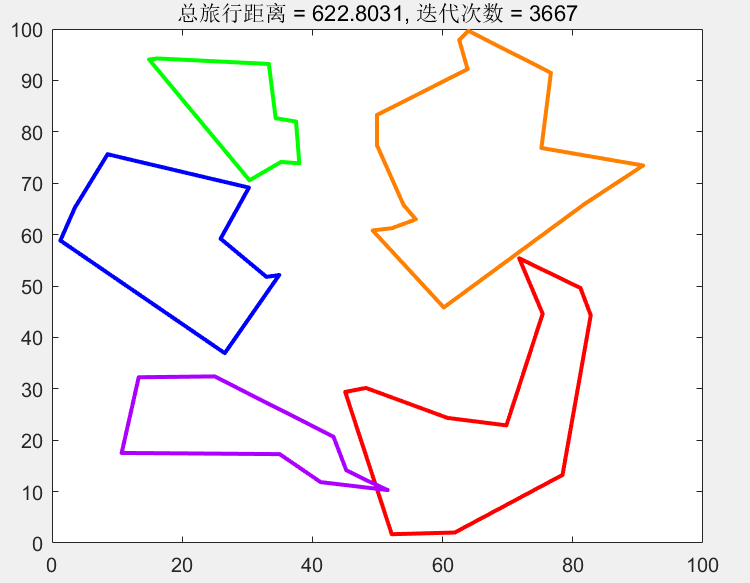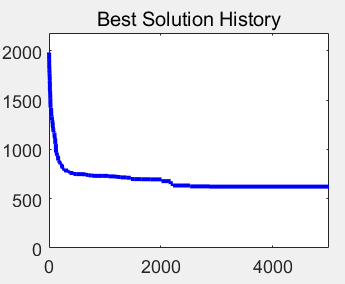若有小伙伴对机器人任务分配算法较为兴趣的，可以在下面评论交流一波，纯属互相学习!
展开全文matlab python 机器学习 推荐算法 聚类
• 遗传算法 MTSPMTSP求解处理快递运输最优化问题
• 多旅行商问题，主要解决多条路线且路线路程之和最短问题
• 多旅行商解决单起点闭回路的问题，matlab解决简单简单方便。python 有问必答...